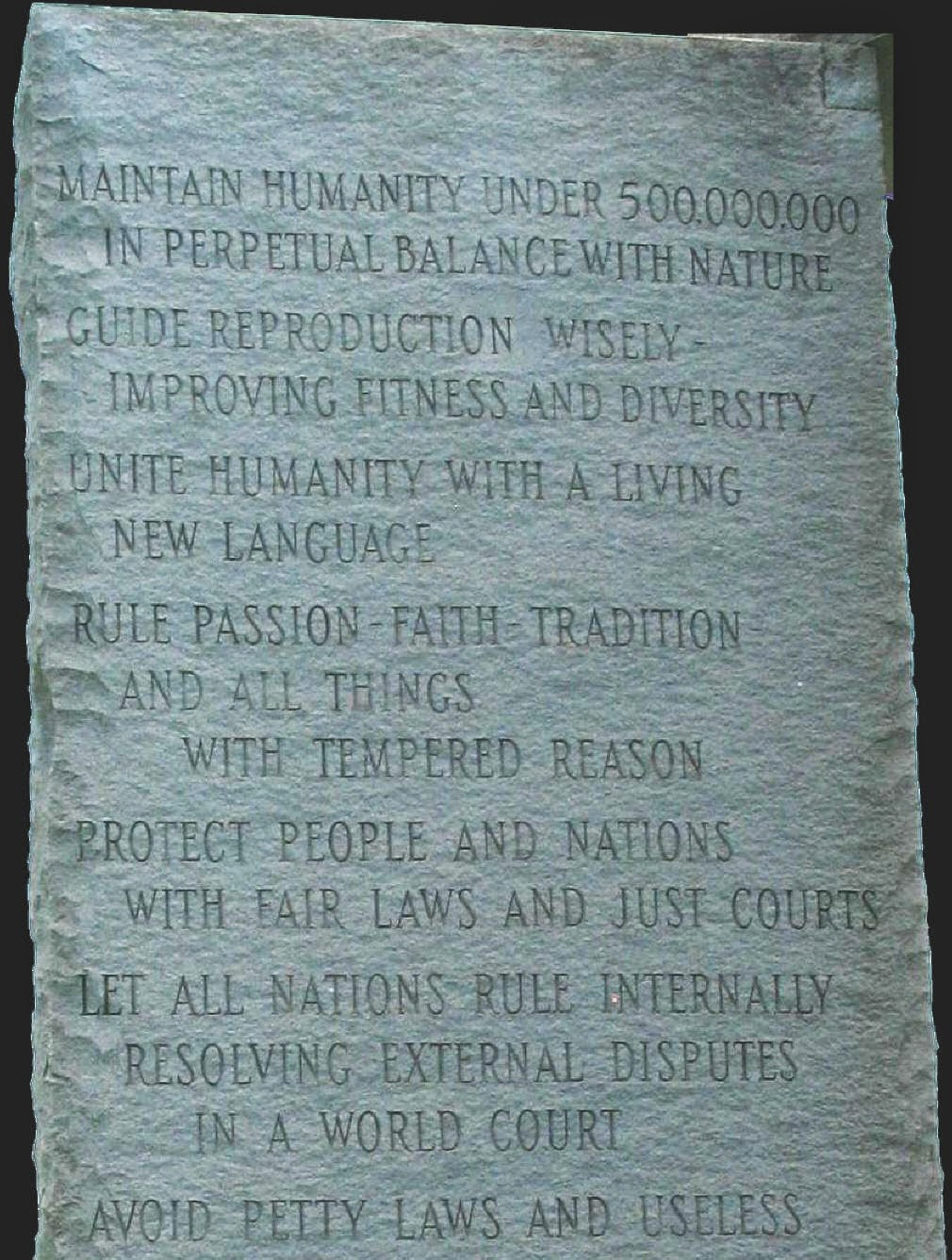## Tuesday, April 22, 2014

### 26 | Faith and God Numerology and 44 | Faith and Hope NumerologyGeorgia Guidestones, March 22, 1980-Present

To believe in God, you must have faith.
• God = 7+15+4 = 26
• Faith = 6+1+9+2+8 = 26
It is a sin to worship a flag.
• Flag = 6+12+1+7 = 26
Some say God is for the sheep.
• God = 7+15+4 = 26
• Sheep = 1+8+5+5+7 = 26
There are phrases such as "cosmic coincidence" to explain the inexplicable.
• Cosmic = 3+6+1+4+9+3 = 26
By the looks of magazines, it seems we worship "royalty" instead of God.
• Royal = 9+6+7+1+3 = 26
Notice the 96, the number of Satanism, the opposite of Yin-Yang, 69, balance.
• Satanism = 19+1+20+1+14+9+19+13 = 96
The way the majority of the world is ruled today, is rooted in historic royalty of England and the U.K.  That is why English has become the language of the world.  If you've read the Georgia Guidestones,  you might recall the third rule of the ten posted, following 1:  Maintain the earth's population at 500million  2:  Guide reproduction wisely  3:  Unite the world under a new living language.  English is that "new living language".  Check this out...

In numerology, 55 and 10 are considered the 'God' numbers; this is because 1+2+3+4+5+6+7+8+9+10 = 55.  In numerology, 55 reduces to 10 because 55 = 5+5 = 10.  Thus the number 10 is a reflection of itself and all that came before it.  With that in mind, let us look at the following words.
• Heaven = 8+5+1+22+5+14 = 55
• Sky = 19+11+25 = 55
• Cloud = 3+12+15+21+4 = 55
• Satan = 19+1+20+1+14 = 55
Think about that, Satan = 55, the God Number.  Take a second look at your currency and ask yourself, "In what God do we trust?"  Also think about this, God and "lie" have the same numerology.
• God = 7+15+4 = 26
• Lie = 12+9+5 = 26

The powers that be, they're laughing at us.  Laughing at us!  And if you ever wonder how Madonna went from homeless to... Madonna, check it out.
• Madonna = 4+1+4+6+5+5+1 = 26
It should be noted, when you sum faith with the standard method, you get the output of 44.
• Faith = 6+1+9+20+8 = 44
This matches the numerology of "hope", another act of dis-empowerment many people make  the mistake of doing on a regular basis.  The 44th President, Barakc Hussein Obama ran on "hope".
• Hope = 8+15+16+5 = 44
The numerology of 44 is associated with other words of destruction.
• Kill = 11+9+12+12 = 44
• Cook = 3+15+15+11 = 44
• Military = 4+9+3+9+2+1+9+7 = 44

That is why at the end of the day, it is better to believe.
• Believe = 2+5+3+9+5+4+5 = 33
• People = 7+5+6+7+3+5 = 33
• Person = 7+5+9+1+6+5 = 33